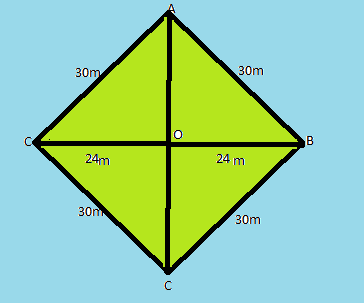QUESTION

# A rhombus shaped field has green grass for 18 cows to graze. If each side of the rhombus is 30 m and its longer diagonal is 48 m (as shown in the figure below the question) , how much area of the grass field will each cow be getting?Hint: In above question first of all we will find the second diagonal of the rhombus field using Pythagoras theorem and and we will use the formula of area of rhombus to calculate its area then by using unitary method we will calculate the area of the grass each cow getting. Also we will use the formula of area of rhombus which is given below,
$area=\dfrac{1}{2}\times {{d}_{1}}\times {{d}_{2}}$ where d1 and d2 are diagonal of the rhombus.

So, in $\vartriangle AOC$ as shown in the figure we have ,
\begin{align} & O{{A}^{2}}={{30}^{2}}-{{24}^{2}} \\ & \Rightarrow OA=\sqrt{900-576} \\ & \Rightarrow OA=\sqrt{324}=18m \\ \end{align}
$area=\dfrac{1}{2}\times 48\times 36=864{{m}^{2}}$
For the 1 cow the area = $\dfrac{864}{18}=48{{m}^{2}}$
Therefore, we get the area of grass field for each cow is equal to $48{{m}^{2}}.$